# Understanding the Discrete Cosine Transform¶

A simple vectorized DCT is implemented and several examples are given that illustrate its operation. Manipulations of the underlying spatial structure that is represented by the DCT are given along with some useful results. The goal is to develop a deeper understanding of the DCT coefficients and how they are used to represent images, since the equation itself is quite opaque.

## DCT Equations¶

The Discrete Cosine Transform expresses a sampled function in the spatial domain as coefficients of cosine wave harmonics (cosines at specific frequencies). The cosines are normalized to express the real part of the orthonormal fourier transform. This allows arbitrary functions to be expressed exactly. No information is lost by taking the DCT and the energy is compacted into the top left corner of the transform, making it a natural starting point for image compression. The entry in the top left corner is termed the DC coefficient, it represents a constant value over the entire image. The other entries are termed AC coefficients.

Give an image (sampled 2D function), the coeffiecient at position $i, j$ is given by

$$D(i,j) = \frac{1}{\sqrt{2N}}C(i)C(j)\sum_{x=0}^{N-1}\sum_{y=0}^{N-1} I(x,y)\cos\left({\frac{(2x+1)i\pi}{2N}}\right)\cos\left({\frac{(2y+1)j\pi}{2N}}\right)$$$$C(k) = \left\{\begin{array}{lr} \frac{1}{\sqrt{2}} & k = 0 \\ 1 & k \neq 0 \end{array}\right.$$

The Inverse DCT is given by

$$I(x,y) = \frac{1}{\sqrt{2N}}\sum_{i=0}^{N-1}\sum_{j=0}^{N-1}C(i)C(j)D(i,j)\cos\left({\frac{(2x+1)i\pi}{2N}}\right)\cos\left({\frac{(2y+1)j\pi}{2N}}\right)$$

## Boilerplate¶

Imports, pretty printing for matrices, colormapping images, etc. This section can be ignored if desired.

In :
!pip install tabulate
!apt-get install -yq ffmpeg

import numpy as np
import scipy.fftpack
import scipy.signal
from IPython.display import display, HTML
from tabulate import tabulate

%matplotlib inline
import matplotlib.pyplot as plt
import matplotlib.image as mpimg
from matplotlib.colors import LinearSegmentedColormap
from matplotlib import animation, rc

np.warnings.filterwarnings('ignore')

def show_mat(m):
latex = tabulate(m, tablefmt="html",floatfmt=".3f")
display(HTML(data=latex))

def show_image(m, ax=None):
c_img = np.zeros((m.shape, m.shape, 3))

max_gr0 = np.max(m[m > 0])

if len(m[m < 0]) > 0:
min_le0 = np.min(m[m < 0])
else:
min_le0 = 0

c_img[m < 0] = np.array([[0.0, 1.0, 0.0]]) * (m[m < 0] / min_le0).reshape(-1, 1)
c_img[m == 0] = np.array([0.0, 0.0, 1.0])
c_img[m > 0] = np.array([[1.0, 0.0, 0.0]]) * (m[m > 0] / max_gr0).reshape(-1, 1)

plt.grid(False)

if ax is None:
return plt.imshow(c_img)
else:
ax.grid(False)
return ax.imshow(c_img)

def show_function(f):
y = np.linspace(0,8,100)
hy = f(y)

plt.xlim(0, 8)
plt.xlabel('Spatial Position')
plt.ylabel('Intensity')
p = plt.plot(y, hy)
plt.show()
return p

Requirement already satisfied: tabulate in /usr/local/lib/python3.6/dist-packages (0.8.2)
Reading package lists...
Building dependency tree...
Reading state information...
ffmpeg is already the newest version (7:3.4.4-0ubuntu0.18.04.1).
0 upgraded, 0 newly installed, 0 to remove and 8 not upgraded.


## DCT Implementation¶

These helper functions compute the normalization and consine harmonics for $N$ spatial frequencies in two dimensions. The function normalize(N) computes the normalizing scale factor $C(k)$ from the above equations. The function harmonics(N) precomputes the cosine values for the $N$ spatial frequences.

In :
def normalize(N):
n = np.ones((N, 1))
n[0, 0] = 1 / np.sqrt(2)
return (n @ n.T)

def harmonics(N):
spatial = np.arange(N).reshape((N, 1))
spectral = np.arange(N).reshape((1, N))

spatial = 2 * spatial + 1
spectral = (spectral * np.pi) / (2 * N)

return np.cos(spatial @ spectral)


The DCT implementation in two dimensions is then very simple in vectorized form. The inverse DCT is also implemented.

In :
def dct(im):
N = im.shape

n = normalize(N)
h = harmonics(N)

coeff = (1 / np.sqrt(2 * N)) * n * (h.T @ im @ h)

return coeff

def idct(coeff):
N = coeff.shape

n = normalize(N)
h = harmonics(N)

im = (1 / np.sqrt(2 * N)) * (h @ (n * coeff) @ h.T)

return im


The following is an example matrix taken directly from the wikipedia JPEG article to show that the implementation above works, it can be found at https://en.wikipedia.org/wiki/JPEG#Discrete_cosine_transform

In :
im = np.array([[-76, -73, -67, -62, -58, -67, -64, -55],
[-65, -69, -73, -38, -19, -43, -59, -56],
[-66, -69, -60, -15, +16, -24, -62, -55],
[-65, -70, -57, - 6, +26, -22, -58, -59],
[-61, -67, -60, -24, - 2, -40, -60, -58],
[-49, -63, -68, -58, -51, -60, -70, -53],
[-43, -57, -64, -69, -73, -67, -63, -45],
[-41, -49, -59, -60, -63, -52, -50, -34]])

dim = dct(im)
idim = idct(dim)

display('Input')
show_mat(im)

display('DCT')
show_mat(dim)

display('IDCT')
show_mat(idim)

'Input'
 -76 -73 -67 -62 -58 -67 -64 -55 -65 -69 -73 -38 -19 -43 -59 -56 -66 -69 -60 -15 16 -24 -62 -55 -65 -70 -57 -6 26 -22 -58 -59 -61 -67 -60 -24 -2 -40 -60 -58 -49 -63 -68 -58 -51 -60 -70 -53 -43 -57 -64 -69 -73 -67 -63 -45 -41 -49 -59 -60 -63 -52 -50 -34
'DCT'
 -415.375 -30.186 -61.197 27.239 56.125 -20.095 -2.388 0.462 4.466 -21.857 -60.758 10.254 13.145 -7.087 -8.535 4.877 -46.834 7.371 77.129 -24.562 -28.912 9.934 5.417 -5.649 -48.535 12.068 34.1 -14.759 -10.241 6.296 1.831 1.946 12.125 -6.553 -13.196 -3.951 -1.875 1.745 -2.787 3.135 -7.735 2.905 2.38 -5.939 -2.378 0.941 4.304 1.849 -1.031 0.183 0.417 -2.416 -0.878 -3.019 4.121 -0.662 -0.165 0.142 -1.072 -4.193 -1.17 -0.098 0.501 1.675
'IDCT'
 -76 -73 -67 -62 -58 -67 -64 -55 -65 -69 -73 -38 -19 -43 -59 -56 -66 -69 -60 -15 16 -24 -62 -55 -65 -70 -57 -6 26 -22 -58 -59 -61 -67 -60 -24 -2 -40 -60 -58 -49 -63 -68 -58 -51 -60 -70 -53 -43 -57 -64 -69 -73 -67 -63 -45 -41 -49 -59 -60 -63 -52 -50 -34

## Understanding¶

The DCT equation is dense and untuitive, but its structure can be understood through examples. We start with simple constant images and move to more complex waveforms, finally showing how arbitrary patterns can be expressed using the transform.

### Constants¶

The first example sets the entire $8 \times 8$ block to ones. This results in only the DC coefficient (the constant term) being set to 8. To understand this, examine the above equation for the IDCT. Only the coefficient in position $(0,0)$ is set and its value is 8. This means that for each pixel, the value is given by

\begin{align} I(x,y) = \frac{1}{4} \cdot C(0) \cdot C(0) \cdot 8 \cdot \cos(0) \cdot \cos(0) = \\ \frac{1}{4} \cdot \frac{1}{\sqrt{2}} \cdot \frac{1}{\sqrt{2}} \cdot 8 = \\ \frac{1}{4} \cdot \frac{1}{2} \cdot 8 = \\ \frac{1}{8} \cdot 8 = 1 \end{align}
In :
all_ones = np.ones((8, 8))

dct_ones = dct(all_ones)
idct_ones = idct(dct_ones)

display('DCT All Ones')
show_mat(dct_ones)

display('IDCT All Ones')
show_mat(idct_ones)

'DCT All Ones'
 8 0 -0 0 0 0 -0 -0 0 0 -0 0 0 0 -0 -0 -0 -0 0 -0 -0 -0 0 0 0 0 -0 0 0 0 -0 -0 0 0 -0 0 0 0 -0 -0 0 0 -0 0 0 0 -0 -0 -0 -0 0 -0 -0 -0 0 0 -0 -0 0 -0 -0 -0 0 0
'IDCT All Ones'
 1 1 1 1 1 1 1 1 1 1 1 1 1 1 1 1 1 1 1 1 1 1 1 1 1 1 1 1 1 1 1 1 1 1 1 1 1 1 1 1 1 1 1 1 1 1 1 1 1 1 1 1 1 1 1 1 1 1 1 1 1 1 1 1

We can perform some simple manipulations on this constant image, for example, suppose we multiply the image by a constant, say 10, and observe the effect on the DCT coefficients.

In :
all_tens = all_ones * 10
dct_tens = dct(all_tens)

display('DCT All Tens')
show_mat(dct_tens)

'DCT All Tens'
 80 0 -0 0 0 0 -0 -0 0 0 -0 0 0 0 -0 -0 -0 -0 0 -0 -0 -0 0 0 0 0 -0 0 0 0 -0 -0 0 0 -0 0 0 0 -0 -0 0 -0 -0 0 0 0 -0 -0 -0 -0 0 -0 -0 -0 0 0 -0 -0 0 -0 -0 -0 0 0

The DC term is now equal to 80, unsurprisingly, this is 10 times the DCT of the ones image. This is due to the linearity of the DCT, e.g:

$$DCT(\alpha \cdot I) = \alpha \cdot DCT(I)$$

This observation has some important practical considerations. For a simple example, consider the above image. To produce the 10s image from the 1s image, we had to multiply all $N \times N$ components by 10. To produce the 10s DCT, we only need to multiply a single time.

Also note that the DC term is exactly 8 times the mean of the original image, and since all of the AC coefficents are 0, they do not depend on the mean at all. This will be extremely important for later work. All of the results in this section hold for non-constant images as well, e.g images with non-zero AC coefficients.

### First Spatial Frequencies¶

Now we can examine the form of the spatial components. Suppose we start with the DCT coefficients and set the coefficient at $(0,1)$ to 1 and the rest to 0. Then we examine the spatial domain image this represents. This is hard to visualize by examining the values of the resulting matrix, so instead we show it as an image.

In all images from here on, red regions are greater than zero, green regions are less than zero, and blue regions are exactly zero. Darker red and darker green are close to zero, lighter red and green are far from zero.

Notice that by setting the first column to 1, we have made a series of small steps horizontally from a maximum value to a minimum value. The first column is the first spatial frequency in the $x$ axis so this makes sense.

In :
dct_01 = np.zeros((8, 8))
dct_01[0, 1] = 1

im_01 = idct(dct_01)
show_image(im_01)
plt.title('First Horizontal Spatial Frequency');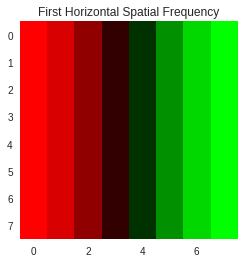Next we do the same for the component at $(1, 0)$, note that we have the same pattern as the previous example, but arranged vertically. Similar to in the previous example, this is the first spatial frequency along the $y$ axis.

In :
dct_10 = np.zeros((8, 8))
dct_10[1, 0] = 1

im_10 = idct(dct_10)
show_image(im_10)
plt.title('First Vertical Spatial Frequency');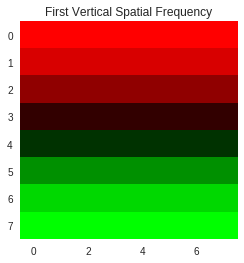Since this is a single spatial frequency, it can also be visualized in one dimension. Note that the expression used for the harmonic definition is just the IDCT equation with the appropriate values plugged in and simplified. Since most of the components are zero it has a very simple form.

In :
def first_harmonic_1d(y):
return (1 / 4) * (1 / np.sqrt(2)) * np.cos(((2 * y + 1) * 1 * np.pi) / 16)

plt.title('First Harmonic (Continuous)')
show_function(first_harmonic_1d);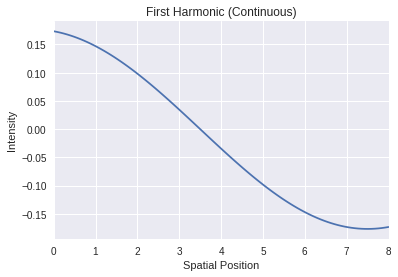This shows that we have a smooth wave with one period equal to the full length of the image. This wave is descritized as part of the transform, leading to the steps in the visualized image.

### Second Spatial Frequencies¶

As we did with the first spatial frequencies, lets examine the second spatial frequency.

In :
dct_20 = np.zeros((8, 8))
dct_20[2, 0] = 1

im_20 = idct(dct_20)
show_image(im_20)
plt.title('2nd Vertical Spatial Frequency')

Out:
Text(0.5,1,'2nd Vertical Spatial Frequency')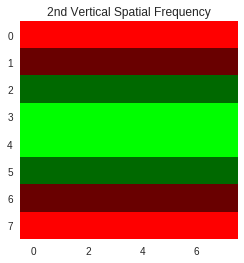This image is more interesting, with large values on the top and bottom and a negative region in the center. Lets again look at the resulting waveform.

In :
def second_harmonic_1d(y):
return (1 / 4) * (1 / np.sqrt(2)) * np.cos(((2 * y + 1) * 2 * np.pi) / 16)

plt.title('2nd Harmonic (Continuous)')
show_function(second_harmonic_1d);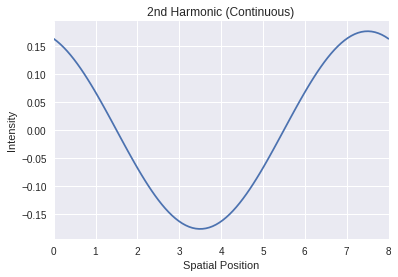So we have a cosine wave that makes (approximately) one and one half periods over the course of the image. Examining the DCT equations, this makes sense. Since we multiply by $i$ or $j$ inside the cosine, this changes the frequency of the wave, so as we move further from the DC coefficient, the cosine completes more periods over the space of the image.

### Last Spatial Frequency¶

Lets contiue this by observing the effect of the final spatial frequency

In :
dct_70 = np.zeros((8, 8))
dct_70[7, 0] = 1

im_70 = idct(dct_70)

plt.figure(figsize=(10, 5))
plt.subplot(1, 2, 1)
plt.title('Last Vertical Spatial Frequency')

show_image(im_70)

def last_harmonic_1d(y):
return (1 / 4) * (1 / np.sqrt(2)) * np.cos(((2 * y + 1) * 7 * np.pi) / 16)

plt.subplot(1, 2, 2)
plt.title('Last Harmonic (Continuous)')
show_function(last_harmonic_1d);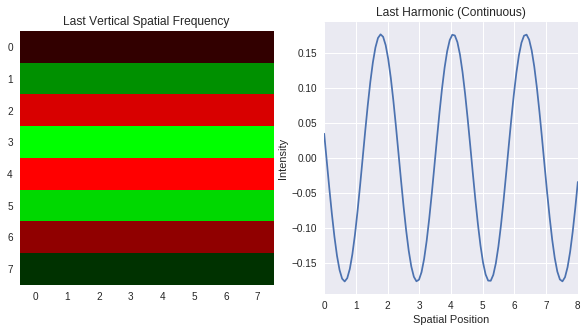You can see that the intensity now varies for every row in the image, and the 1D plot illustrates this as well. Continuting this line of reasoning, the last spatal frequency in both row and column (e.g. position $(7,7)$, the coefficient diagonal to and furthest from the DC coefficient) can set a different value for each individual pixel.

By using these spatial frequencies in linear combinations together with the appropriate weights, any arrangement of pixels can be represented. This is the fundamental idea behind the JPEG image codec.

### Complex Patterns¶

Here are some more complex examples. First, we set the coefficient at $(1, 1)$ to 1. This uses both horizontal and vertical spatial frequencies so it isn't possible to visualize it with a 1D graph.

In :
dct_11 = np.zeros((8, 8))
dct_11[1, 1] = 1

im_11 = idct(dct_11)

show_image(im_11)
plt.title('Sum of 1st Horizontal and Vertical Spatial Frequencies');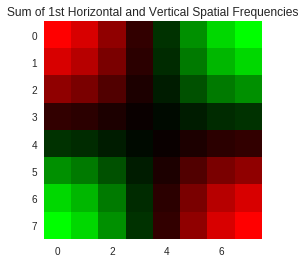Next, something more practical

In :
h_dct = np.zeros((8, 8))
h_dct[:, 0] = [2.250, 0.069, -0.327, -0.196, 0.250, 0.294, -0.135, -0.347]
h_dct[:, 2] = [-1.409, -0.090, 0.427, 0.257, -0.327, -0.384, 0.177, 0.453]
h_dct[:, 4] = [-1.750, 0.069, -0.327, -0.196, 0.250, 0.294, -0.135, -0.347]
h_dct[:, 6] = [2.478, -0.037, 0.177, 0.106, -0.135, -0.159, 0.073, 0.188]

h_char = idct(h_dct).round(3)
show_image(h_char)
plt.title('Image of an H from DCT Coefficients');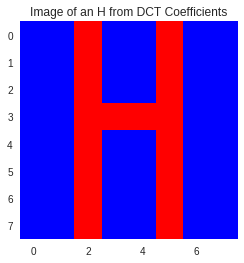Note that in the last example, we round to avoid floating point errors which only make the display look ugly, in practice their contribution is minimal.

Observe that the largest (in magnitude) components of the DCT matrix are in the top row (the first entry in each list), this is the "energy compaction" property of the DCT at work. As you move further from the DC coefficient, the values tend to become smaller.

This last example showed how complex and arbitrary patterns can be made by combining simple consine waves at different frequencies, it is easier to visualize with an animation.

In :
fig, ax = plt.subplots()
ax.set_title('Reconstructing the H Image from Individual DCT Coefficients')

h_anim = np.zeros((8, 8))

imflip = ax.imshow(h_anim)

def init():
imflip.set_data(idct(h_anim))
return (imflip,)

def animate(i):
if i < 32:
non_zeros = np.where(h_dct != 0)
a = non_zeros[i]
b = non_zeros[i]

h_anim[a, b] = h_dct[a, b]
imflip = show_image(idct(h_anim).round(3), ax)
return (imflip,)
else:
imflip = show_image(idct(h_anim).round(3), ax)
return (imflip,)

anim = animation.FuncAnimation(fig, animate, init_func=init,
frames=50, interval=1000, blit=True)

display(HTML(anim.to_html5_video()))

plt.ioff()
plt.close()


In the animation above, each spatial frequency is added in with its appropriate weight one at a time until the final image is completed. Note how the patterns start simple and gradually get more complex finally converging on the H image.

## Why Should This Work?¶

The goal of these notes was the develop a feeling of the DCT by example. By now it may be clear that the DCT does work and how linear combinations of simple waveforms can sum to complex patterns, but the question of why it should work at all remains unanswered. This is tied to the fundamental result of the Fourier transform. This idea is presented here briefly for completeness and without proof or formal derivation.

### Orthonormal Vectors and the Standard Basis¶

Consider the arbitrary 2D vector $[5, 3]$. It is commonly thought of as being a point plotted on a 2D axis. One axis is termed $x$ and the other $y$. If we want to refer to the distance along each axis, we say that the $x$-coordinate is 5 and the $y$-coordinate is 3. This is a fundamental convention of linear algebra. Another fundamental idea in linear algebra is that these vectors can be represented as a linear combination of some standard basis. In 2D, this standard basis is the set $\{[1, 0], [0, 1] \}$. Then in our example, we can say that $[5, 3] = 5[1, 0] + 3[0, 1]$.

What makes this standard basis special? Aside from the simple form of the vectors in the basis, there are two properties. The first is that the vectors are orthogonal. Two vectors are considered orthgonal if their inner product is 0. So $[1, 0] \cdot [0, 1] = 1\cdot0 + 0\cdot1 = 0$. Orthogonal vectors are desirable because they represent quantities along decoupled dimensions. For the standard basis, those directions were of the $x$ and $y$ axes. The second property is that the vectors are normalized, e.g. their magnitude is 1. The magnitude of a vector is given by its $l_2$ norm: $|x|_2 = \sqrt{\sum_i x_i^2}$. For the same standard basis we have been considering this gives $\sqrt{0^2 + 1^2} = \sqrt{1^2} = 1$. This is desirable because there are no extra scale factors in the basis vectors, any scaling is done only be the magnitude of the vector we are trying to represent.

Given a set of vectors, if each of the vectors are normal and they are all orthogonal, then we call the set orthonormal. Provided there are as many vectors as dimensions, then this set is a good choice for a basis. Note that besides the standard basis, there are an infinite number of other orthonormal bases in 2D and any of them could be used instead of the standard basis. The standard basis is considered easy to work with because it contains only 1s and 0s. Nothing in this section should be considered news, but the basic ideas will be built upon in the next sections.

### Infinite Dimensional Spaces¶

In the previous sections, we considered 2D vectors, but this obviously works for 3, 4, ... dimensions. But what about an infinite number of dimensions? Perhaps surprisingly, even for an uncountable number of dimensions, the ideas in the previous section still hold. What does an infinite dimensional vector look like? How can we even work with such quantities? Consider a vector $x$ of finite dimension. We say that the $i$th component of $x$ is $x_i$. This is purely notational, and there is no reason why this notation should fail us when we extend to infinite dimensional spaces. The main problem is that while we can write down the list of numbers that a finite dimensional vector has for example $x = [1, 3, 5, 7]$, we can't write them all down for infinite dimensional spaces. What if we had, instead, a rule to express the vectors values. For example, with $x$, we would have $x_i = 2i - 1$ for $i \in [0, 3]$. This would allow us to retrieve the value of an infinite dimensional vector, given that we can write down such a rule for it. So extending $x$ to the infinite dimensional space, we have $x_i = 2i - 1$ for $i \in \mathbb{R}$, this is a valid point in an infinite dimensional space. In mathematics, there is another name for the rule of $x_i$: it is a function. This is a fundamental result that Fourier used: all functions can be thought of a points in an infinite dimensional space. For another example consider the function $f(k) = \log(k)$. Instead of thinking of $k$ as the independant variable input to the $\log$ function, think of it as indexing a vector, The to get the $k$th component of that vector, you compute $\log(k)$, so $\log(k)$ itself is a point in infinite dimensional space.

### An Orthonormal Basis of Infinite Dimensional Space¶

A natural question to have at this point, since we just got done discussing orthonormal bases, is: can that same concept be extended to infinite dimensional space? The answer is yes. However, consider what we would need in order to have such a basis. We need a set of vectors which are all normal and orthogonal to each other. This was easy enough to compute when we had finite vectors, but what about functions? How can a function be normal? How can a function be orthogonal to another function? It's easy if we stop thinking of them only as functions and instead think of them as vectors. Recall that a finite vector $x$ is normal if

$$|x|_2 = \sqrt{\sum_i x_i^2} = 1$$

To extend this to functions, we instead have

$$|f(x)|_2 = \sqrt{\int\limits_{-\infty}^{\infty} f(x)^2 dx} = 1$$

With the integral stepping in as a continuous version of the sum. What about orthogonal functions? For two finite vectors $x$ and $y$, they are orthogonal if

$$x \cdot y = \sum_i x_i y_i = 0$$

You may be able to guess the function form now. Two functions $f(x)$ and $g(x)$ are orthogonal if

$$f(x) \cdot g(x) = \int\limits_{-\infty}^{\infty} f(x)g(x) dx = 0$$

So if we can find a set of functions that fit these criteria then we have found an orthonormal basis for infinite dimensional space.

### A Simple Solution¶

Consider, for no particular reason, the functions $\sin(x)$ and $\cos(x)$. Also for the sake of simplification, lets restrict the domain of these functions to the interval $[-\pi, \pi]$ (they are periodic anyway). Let's check if these functions are orthogonal:

$$\int\limits_{-\pi}^{\pi} \cos(x)\sin(x) dx$$

This integral can be solved quite easily by substitution: let $u = \cos(x)$ and $du = -\sin(x) dx$. then we have

$$\int -u du \\ = - \int u du \\ = -\frac{u^2}{2}$$

Substituting back in:

$$-\frac{u^2}{2} = -\frac{\cos^2(x)}{2} \Biggr|_{-\pi}^{\pi} \\ = -\frac{\cos^2(-\pi)}{2} + \frac{\cos^2(\pi)}{2} = 0$$

So $\sin(x)$ and $\cos(x)$ are orthogonal functions. Are they also normal? (This can be solved using trigonometric identities, the solution is skipped)

$$\int\limits_{-\pi}^{\pi} sin^2(x) dx = \pi$$

Convince yourself that this is also true for $\cos(x)$. While they are not normal, they are within a constant of being normal. So the two functions $\frac{cos(x)}{\pi}$ and $\frac{sin(x)}{\pi}$ are orthonormal. This means that they are an orthonormal basis for functions.

### The Fourier Transform¶

There are a few oversimplifications in the last section, for example the domain is restricted and there are only two functions given when really we are looking for an infinite set of functions. That section layed out only the basic building blocks, a full derivation is beyond the scope of the document. The actual fourier transform of a function is given as

$$\hat{f}(\zeta) = \int\limits_{-\infty}^{\infty} f(x) e^{-2\pi i x \zeta} dx$$

Which looks intimidating but really it is just the same $\sin(x)$ and $\cos(x)$ from the last section combined using Euler's formula into a single $e^x$. This also gives the infinite family of cosines and sines at different frequencies that we needed. As in the first part of this section, where the 2D vector was written as a linear combination of the basis vectors, this formula expresses a function a linear combination of the infinite basis vectors. In my opinion, it is not so important to understand every detail of this equation, as it is rather opaque. The important thing to understand is the logic of where it came from and the idea of functions being points in an infinite dimensional space.

### Closing Remarks¶

First off, why would we care about this? Besides being interesting, it allows any function, even a function we don't really know the form of to be expressed as a linear combination of sines and cosines. These are functions that we can do math on: they are well studied. Furthermore, it has myriad use in signal processing to understand the the components, or harmonics which make up a complex waveform.

So what about the DCT? Take the real part of a Fourier transform and you get the cosine transform (since the real part only includes cosines). Descritize that transform so that it works on impulses, or functions with a single value in their domain, and you get the Discrete Cosine Transform. The DCT that we used for most of this document contains extra scale factors to ensure that the transform is orthonormal.

© 2018 Max Ehrlich